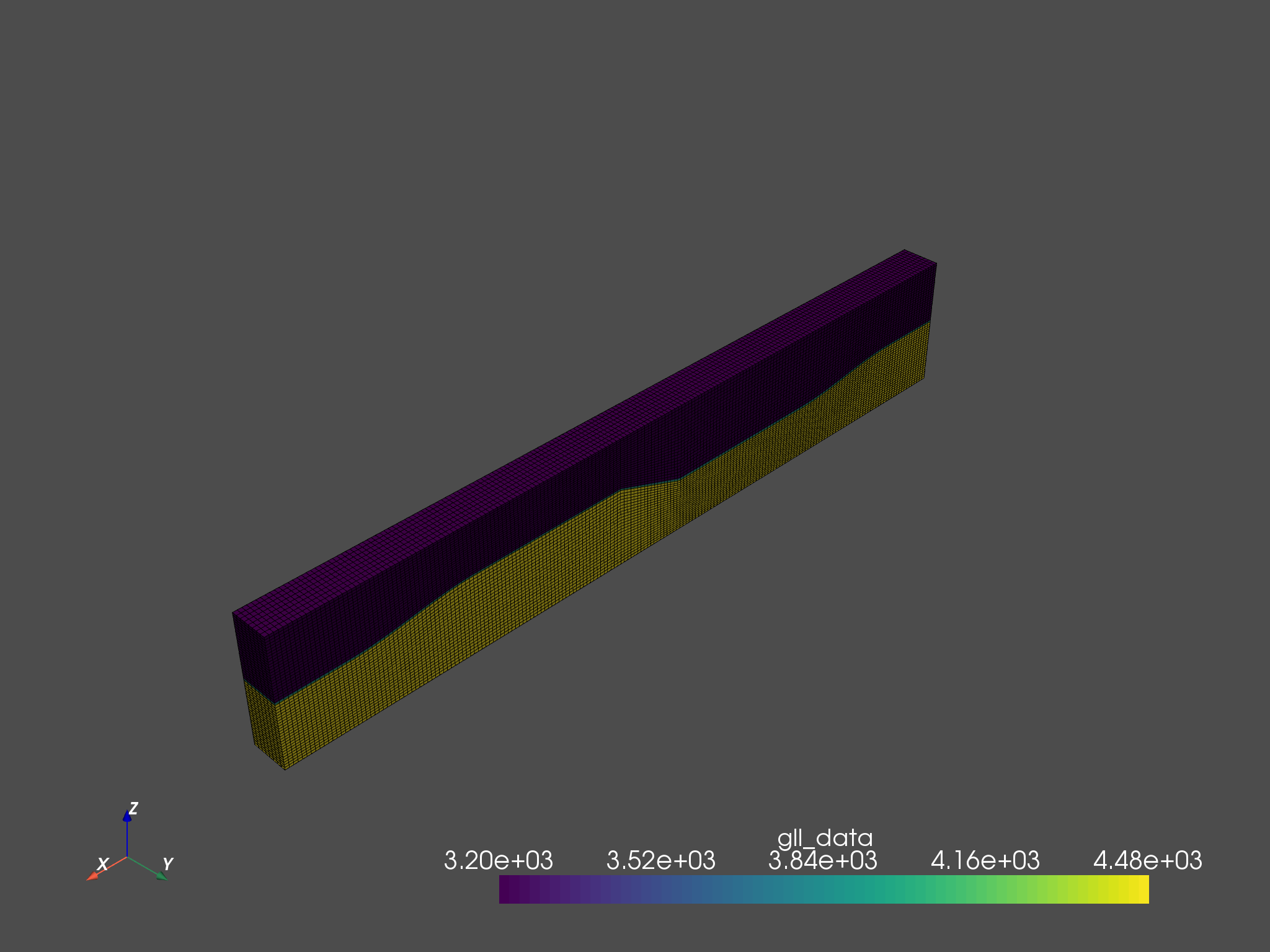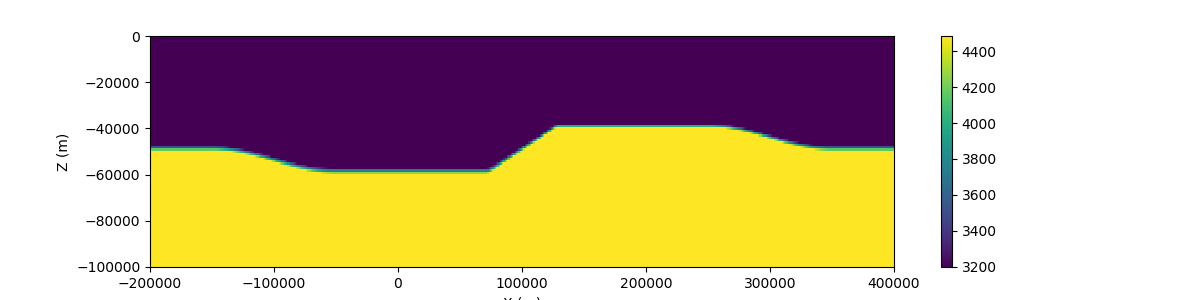VTK是用于三维可视化的重要格式。如果我们要对三维可视化数据进行更多自定义的计算，则需要将其转化为适用与科学计算的数据格式。 （此图由PyVista生成）

## 1. 读取并拼合所有VTK文件

VTK文件通常生成与大型并行计算程序，程序有时会将整个网格区域划分为多个子区域进行分布式计算。所以在读取网格同时也要将所有网格拼合在一起，组成完整的网格。我们使用PyVista模块来进行该处理。首先导入所需模块

 1 2 3  import numpy as np import glob import pyvista as pv

### 循环读取每个VTK文件

 1  blocks = [pv.read(fname) for fname in sorted(glob.glob('*.vtk'))]

### 拼合所有区块

PyVista提供了MultiBlock用于拼合不同区块，输出的meshUnstructuredGrid类型，可以用于后续处理

 1 2  points_vtk = pv.MultiBlock(blocks) mesh = points_vtk.combine()

## 2. 对数据网格化并重采样

 1 2  grid = pv.create_grid(mesh, dimensions=(600, 60, 100)) image = grid.sample(mesh)
• dimensions定义了在X、Y和Z方向的采样点个数，点数越多重采样的精度越接近原始网格。

 1  mat = image.point_arrays['values'].reshape((image.dimensions[::-1]))
• 'values'取决于原始数据的键名，如Specfsem3D生成的网格，数值名为gll_data

 1 2 3  x = np.linspace(image.bounds, image.bounds, image.dimensions) y = np.linspace(image.bounds, image.bounds, image.dimensions) z = np.linspace(image.bounds, image.bounds, image.dimensions)

## 3. 预览重采样后的结果

 1 2 3 4 5 6  import matplotlib.pyplot as plt plt.pcolor(x, z, mat[:, 15, :]) plt.colorbar() plt.ylabel('Z (m)') plt.xlabel('X (m)') plt.show()